# Dynamics Quiz 1

#### Quiz Description

This A level physics quiz is all about Dynamics. Here, we are going to cover some important sections of dynamics such as the application of Newton’s third law, calculation of resultant force, etc. In a nutshell, this quiz covers a great section of dynamics, thus, answering the quiz correctly is a guarantee of your success in answering most dynamic questions correctly.

Dynamics is another branch of mechanics, and it is concerned with the study of forces and their relationship with motion and equilibrium. Unlike kinematics, dynamics is further divided into two types: linear and rotational dynamics. Linear dynamics studies the motion of bodies in a straight line, whereas Rotational dynamics studies the motion of bodies in a circular or curved path. We’ve heard of forces, what is a force?

A force is a push or a pull exerted on a body, and there are two categories of forces which are action-at-a-distance force, and contact force.

Dynamics is a very important topic that should be mastered to the fingertips. For this reason, this quiz, and other A level Physics quizzes are available on this platform for you to train yourself to understand better. Wish you the best as you solve the problems!

A man pulls a sledge of mass 25 kg across level ground with a horizontal force of 60 N.

A constant force of friction of 20 N acts on the sledge.

What is the acceleration of the sledge?

Correct
• 0.63 m / s 2
• 1.6 m / s 2
• 2.4 m / s 2
• 3.2 m / s 2

A car moves in a circle at constant speed.

What is the direction of the resultant force acting on the car?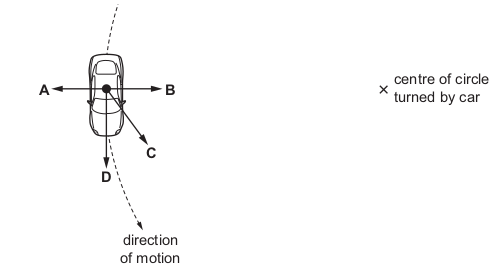Correct
• A
• B
• C
• D

Four forces act at a point as shown.

What is the size of the resultant force?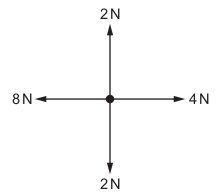Correct
• 0N
• 4N
• 6N
• 8N

When a car turns a corner at speed, it risks toppling over. Two factors affecting the stability of a car are the height of its centre of mass and the distance between its front wheels.

Which factors make the car most stable?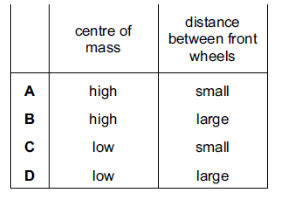Correct
• A
• B
• C
• D

A metal ball of mass 0.30 kg and weight 3.0 N is held so that it is below the surface of oil.

It experiences an upwards force of 0.30 N.

When the ball is released, what is its initial acceleration?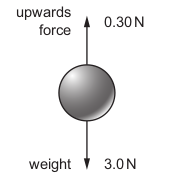Correct
• 1.0 m / s 2
• 9.0 m / s 2
• 10 m / s 2
• 11 m / s 2

The Earth travels in a circular orbit around the Sun at constant speed.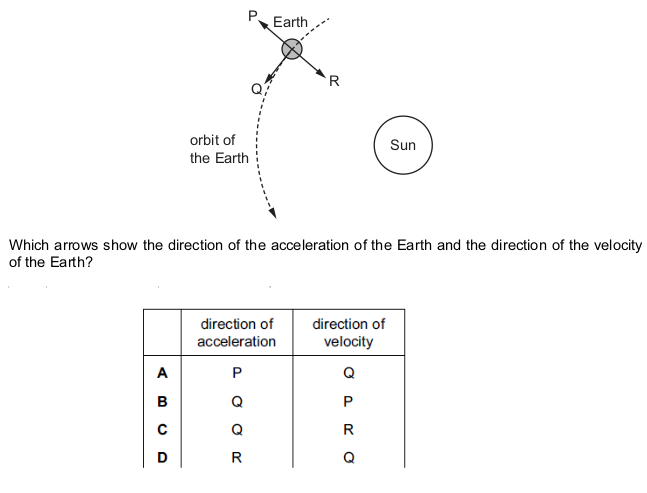Correct
• A
• B
• C
• D

In which example does friction act in the direction of forward motion of the object on which it acts?

Correct
• on a suitcase accelerating on a conveyor belt
• on sandpaper rubbing a wooden block
• on the skis of a skier accelerating downhill on smooth snow
• on the tyres of a braking car

An apple of mass 0.15 kg and weight 1.5 N falls from a tree. At one point during its fall, the air resistance on the apple is 0.60 N upwards.

What is the acceleration of the apple at this point?

Correct
• 4.0 m / s 2
• 6.0 m / s 2
• 10 m / s 2
• 14 m / s 2

Four of the gravitational forces that act between bodies in the Solar System are described below.

P the force on the Moon due to the Earth

Q the force on the Earth due to the Sun

R the force on the Earth due to the Moon

S the force on the Moon due to the Sun

Which two forces are a Newton’s third law pair (action and reaction)?

Correct
• P and Q
• P and R
• P and R
• R and S

A man pushes a heavy box along the ground.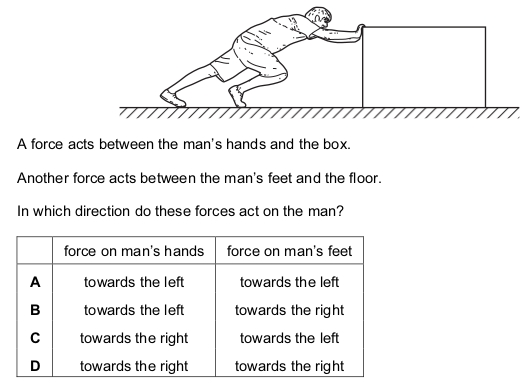Correct
• A
• B
• C
• D

In which situation is the resultant force on the body equal to zero?

Correct
• a car turning a corner at constant speed
• a rock falling freely on the Moon
• a train going up a straight hill at constant speed in a straight line
• an aircraft accelerating along a runway in a straight line

A satellite is orbiting the Earth.

What is the direction of the force on the satellite causing this circular motion?

Correct
• away from the centre of the Earth
• in the direction of motion of the satellite
• in the opposite direction to the motion of the satellite
• towards the centre of the Earth

The diagram shows a block of stone on a rough horizontal surface.

Force P acts on the block as shown.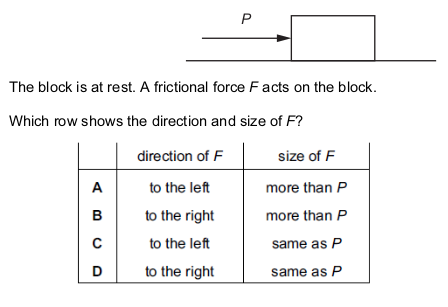Correct
• A
• B
• C
• D

The distance traveled by a car is increasing uniformly as it is driven along a straight road up a hill.

Which quantity for the car is constant but not zero?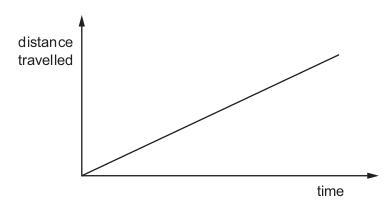Correct
• acceleration
• gravitational potential energy
• kinetic energy
• resultant force

A boy sits on a playground roundabout (carousel). The roundabout carries the boy in a horizontal, anticlockwise circle at a constant speed.

The diagram shows the view of the roundabout from above. What describes the resultant force on the boy?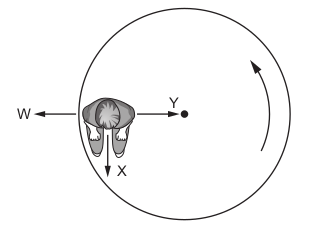Correct
• The resultant force is zero.
• The resultant force is in direction W.
• The resultant force is in direction X.
• The resultant force is in direction Y.

In a model of an atom, electrons move in circular orbits around a nucleus. Which statement about the electrons is correct?

Correct
• The electrostatic force on the electrons is away from the nucleus.
• The acceleration of the electrons is towards the central nucleus.
• The speed of the electrons varies continuously.
• The velocity of the electrons remains constant.

A particle P is moving in a horizontal circle about O. P moves at constant speed. Which statement is true?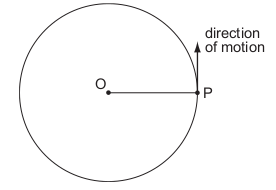Correct
• A force of constant size acts on P in the direction of motion.
• A force of constant size acts on P towards O.
• The force on P varies in size as it moves around the circle.
• There is no resultant force acting on P.

A body slides down a friction-less slope as shown.

As the body presses on the surface, the surface pushes back on the body. The force of the

surface on the body is sometimes called the reaction force.

In which direction does the reaction force act?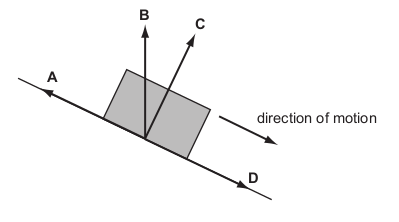Correct
• A
• B
• C
• D

Which object has the largest resultant force?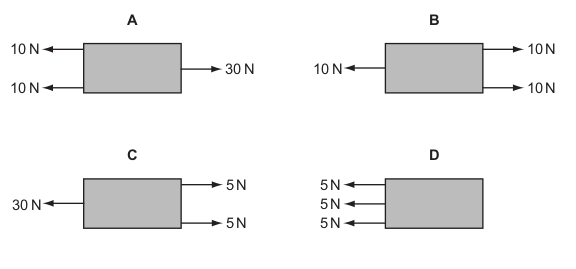Correct
• A
• B
• C
• D

The diagram represents the Moon in its orbit around the Earth.

Which arrow represents the direction of the resultant force acting on the Moon at the instant

shown?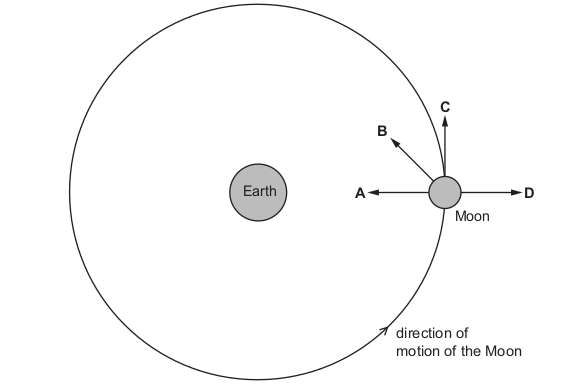Correct
• A
• B
• C
• D